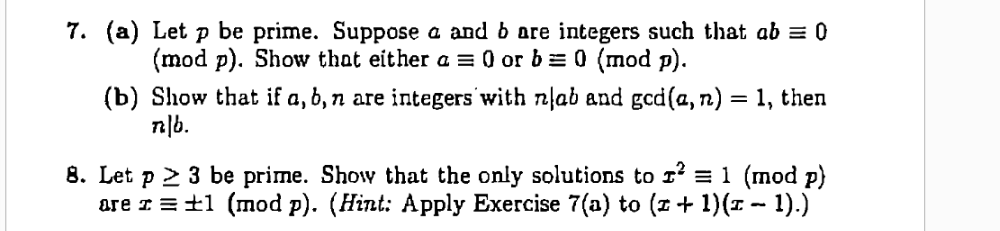# 7. (a) Let p be prime. Suppose a and b are integers such that ab = 0 (mod p). Show that either a = 0 or b = 0 (mod p). (b) Show that if a, 6, n are integers with nlab and gcd(a, n) = 1, then n|b. 8. Let p 2 3 be prime. Show that the only solutions to 1' = 1 (mod p) are I = ±1 (mod p). (Hint: Apply Exercise 7(a) to (z+ 1)(1 - 1).)

Question

please do 8help_outlineImage Transcriptionclose7. (a) Let p be prime. Suppose a and b are integers such that ab = 0 (mod p). Show that either a = 0 or b = 0 (mod p). (b) Show that if a, 6, n are integers with nlab and gcd(a, n) = 1, then n|b. 8. Let p 2 3 be prime. Show that the only solutions to 1' = 1 (mod p) are I = ±1 (mod p). (Hint: Apply Exercise 7(a) to (z+ 1)(1 - 1).) fullscreen

## Expert Answer

### Want to see this answer and more?

Experts are waiting 24/7 to provide step-by-step solutions in as fast as 30 minutes!*

*Response times vary by subject and question complexity. Median response time is 34 minutes and may be longer for new subjects.
Tagged in© 2021 bartleby. All Rights Reserved.